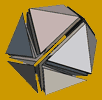theory

# Lattice Point Counting and Integration over Convex Polytopes

The papers below discuss algorithms and software for the main functions of LattE: enumeration of all lattice points inside a rational convex polytope, integration of a polynomial over a polytope, and computing coefficients of Ehrhart quasi-polynomials. The common idea of all our "algebraic-analytic" algorithms consist of using rational generating functions to represent either lattice point sets or integrals of functions over polyhedral regions. These algorithms are implemented in LattE, which was the first computer implementation of A. Barvinok's algorithm for unimodular cone decomposition.

## Lattice Point Counting

The very first complete paper describing LattE is Effective Lattice Point Counting in Rational Convex Polytopes by J. A. De Loera, R. Hemmecke, J. Tauzer, and R. Yoshida, Journal of Symbolic Computation 38 no. 4 (2004) pp. 1273-1302 doi:10.1016/j.jsc.2003.04.003

Some key papers and websites that supported further development are

### Primal Variants of Barvinok's Algorithm

The theory behind the development of LattE macchiato was presented by M. Köppe in

### Application of Algebraic-Symbolic Counting Algorithms in LattE

Professor De Loera presents two interesting applications of counting lattice points:

## Integration

The next two papers explore how to integrate polynomials over polytopes, as implemented in LattE integrale version 1.5.

## Highest Coefficients of Weighted Ehrhart Quasi-Polynomials

The following paper explains how to compute the highest coefficients of weighted Ehrhart quasi-polynomials as step-polynomials, as implemented in LattE integrale version 1.6.

## Research Monograph and Textbook

Algebraic and Geometric Ideas in the Theory of Discrete Optimization (2012, xx + 313 pages) by J.A. De Loera, R. Hemmecke and M. Köppe. This book presents recent advances in the theory of discrete optimization, particularly those arising from algebraic geometry, commutative algebra, convex and discrete geometry. The material within is not yet well known, but it is accessible to students of mathematics, computer science, algorithms or operations research at the advanced undergraduate level and beyond. In particular, part III: Generating Function Methods, deals with many ideas core to the main LattE functions. See the contents for more.• Mathematics • The University of California, Davis •
• One Shields Avenue • Davis, CA 95616 •